# Special Relativity and Newtonian Mechanics beyond the Speed of Light and for Objects with Negative Mass

0
6072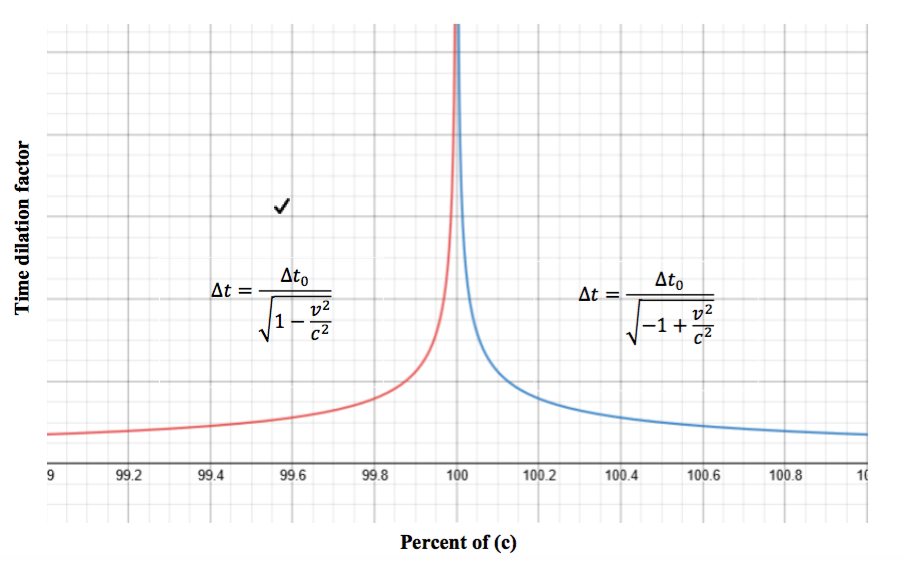Abrar Syed

McLean High School

Contact: abrar2020syed@gmail.com

Peer Reviewer: Cole Johnson

Professional Reviewer: Mohsina Asif

Abstract: The following paper discusses the effects of special relativity and Newtonian mechanics on particles theoretically traveling faster than the speed of light, and for objects with negative mass. The primary focus will be on deriving a new gamma value for Lorentz transformations which works for velocities exceeding the speed of light (c). Later parts of this paper will discuss possible ways in which an object can travel beyond light speed, which involves Bose-Einstein Condensates (BECs), these condensates have been said to exhibit a ‘negative mass’ like behavior. The goal of this paper is not to contradict the current models of special relativity and Newtonian mechanics, but to add to them. Currently, the Lorentz transformation simply does not work for objects exceeding the speed of light, as it will output imaginary values. A new gamma is derived, and works for velocities beyond the speed of light. The fifth part of this paper discusses the possible behavior of negative mass, and how this behavior complies with the current model of Newtonian mechanics. Since the gamma derived in the Lorentz transformation is essential to all other relativistic contractions, this is what will be discussed first. The overall outcome of this paper is to add to the current models of special relativity and Newtonian mechanics.

Part 1: Lorentz Factor and Relativistic Mechanical Quantities

Variables:

Assumptions (classical):

• The speed of light is constant in a vacuum and cannot be reached by a particle with mass
• To compensate for this, other mechanical quantities must change
• Given two frames of references moving at different velocities (one stationary and one non-stationary), their mechanical quantities can be related
• Mass is positive
• Between two reference frames, one moving and one stationary, the following relationships can be established (Galilean Transformations) (HyperPhysics. (n.d.)):

(With this information, the Lorentz transformation can be derived)

The standard derivation is as follows:

• A stationary frame and a moving frame have a point S, where they coincide in time and position
• For the sake of simplicity, the z axis will be omitted, as Minkowski space-time can be drawn with (ct) as the y-axis, and (x) as the x-axis

Stationary frame:

Moving frame

Using the Galilean transformations, and assuming the two frames are related by some factor ?, the following relations are established:

This can be written in terms of ct’ and ct:

The two can be related by solving the top equation for t’ :

This is substituted into the second equation to relate the two reference frames:

Solve for Gamma:

Relativistic Mechanical Quantities (v < c) (current accepted model)

Below are the current relativistic contractions for mechanical quantities such as time, length, mass, and momentum. The equations for other quantities, like energy, will not be discussed, as some do not rely on the use of gamma. The effects of time dilation will be elaborated on in Part 4 of this paper, labeled: Time Dilation. As mentioned above, however, none of these equations work for velocities exceeding the speed of light.

Time:

Length:

Mass:

Momentum:

Part 2: Proposed Lorentz Factor for Objects with Velocity Exceeding the Speed of Light

The following is a derivation of the Lorentz factor, with a few new assumptions regarding the speed of light. The end result will be a gamma which resembles the current one but works for velocities exceeding the speed of light. However, the uniqueness of the speed of light still holds, as this gamma also indicates that the speed of light cannot be attained. Hence, a particle traveling beyond the speed of light will require an infinite amount of energy to slow down: similar to how a particle with a velocity less than the speed of light requires an infinite amount of energy to attain it.

New Assumptions:

• The speed of light is constant in a vacuum
• To ensure that no object can ever reach the speed of light (either by speeding up or slowing down), mechanical quantities must change
• Given two frames of references moving at different velocities (one stationary and one non-stationary), their mechanical quantities can be related
• Mass can be negative, assuming elemental particles related to mass can have various spin states which allow for such properties

The new transformations can be written as:

*Note: Since ct’ must always be positive, given the parameters assumed in the classical derivation, the factor (ctvt) must be written as (vtct), since is greater than c. This does raise the question, however, of what the movement of the particle would look like. That problem is yet to be addressed; however, Part 4 of this paper shows that the gamma which is to be derived satisfies the equation for time dilation, and helps develop a definite limit as approaches c.

The two can be related by solving the top equation for t’ :

This is subbed into the second equation to relate the two reference frames:

Solve for Gamma (which is now denoted as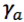to avoid confusion)

Relativistic Mechanical Quantities (v > c) (proposed addition to current model)

Below are the new relativistic contractions for mechanical quantities such as time, length, mass, and momentum. The equations for other quantities, like energy, will not be discussed, as some do not rely on the use of gamma and will be discussed in a later paper. The effects of time dilation will be elaborated on in Part 4 of this paper, labeled: Time Dilation. All of these equations work for objects which have a velocity greater than the speed of light.

Time:

Length:

Mass:

Momentum:

Part 3: Relationship between the Two Gammas

Similarly, given the current gamma, the new one can be derived by multiplying the current gamma by i.

The relationship between the two is simple. Multiplying the original gamma with (i) will result in the new gamma which works when velocity exceeds the speed of light. Hence, all the special relativity equations can be written as a piecewise function.

Part 4: Time Dilation

Below is a proof that time dilation is infinite at the speed of light, which is proven by creating a new equation for velocities exceeding c. A majority of this section is itself a previous incomplete (and unpublished) paper of mine, I saw it fit to put it in this paper, as it reaffirms the new gamma which has been derived.

The following is proof that time dilation at the speed of light is infinite by creating an equation for time dilation beyond the speed of light. With the current equation for time dilation, the left- hand limit as the velocity of an object reaches the speed of light is infinity. However, without the right-hand limit, there is no way to know for sure that time dilation at the speed of light is infinite. The following derivation hopes to create an equation for time dilation that works for objects (theoretically) traveling faster than the speed of light. By doing so, the nature of time dilation at the speed of light (and beyond) is better understood.

Current equation:

• This equation offers no real values for objects traveling faster than the speed of light, as any value exceeding will result in imaginary time dilation.
• And only a left hand limit exists, sodoes not exist.

The following derivation reflects the current derivation of time dilation based on the light clock experiment.

The setup is as follows: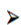A light clock has two mirrors which reflect a beam of light back and forth, the time ittakes for the light to hit one mirror and bounce back is called one “tick”.

(Derivation of Time Dilation. (n.d.).)

• While in the same inertial reference frame as the clock, the time it takes the light to complete one trip is:
• Where ‘d’ is the distance between the mirrors, and ‘c’ is the speed of lightWhen this clock is moving at some velocity ‘v’ (less than the speed of light), and is observed from an outside reference frame, the observed path of the light will look something like this:

This is often drawn as:

However, if a particle were to be moving faster than the speed of light, then the leg of the right triangle “(v)(t) / 2” will become the hypotenuse, as it is greater than L (which is defined as the distance traveled by the particle of light over the observed time interval). Since the observed time for both velocities is the same, and is now greater than c, (v(t)/2) will result in a greater length than c(t). Moreover, the values (d) and (L) cannot change, as the speed of light cannot change, hence the system itself will have to contract in some way (the light clock has essentially flipped).This relationship can be written with the Pythagorean Theorem:Solve for L:This is substituted into the observed time equation: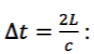With these assumptions, a relationship can be established between a stationary light clock, and one moving beyond the speed of light

Given that: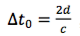and solving for ‘d’ results in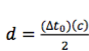, both times (stationary and moving) can be written in the same equation, by substituting for ‘d’ in the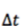equation:

By combining these two equations, a definite limit can be established for when v = c. With the previous equation, the time dilation for values less than the speed of light was indicated, hence resulting in a left-hand limit as v approaches c. With values for time dilation beyond light speed, a right-hand limit also emerges.

Graph of both equations:

(Desmos graph. (n.d.))

When both equations are graphed, it is seen that the derived equation for objects traveling at a speed faster than that of light mirrors the equation used for objects traveling at a speed less than that of light, over the asymptote “= c”. The graph on the left is multiplied by a factor of i to produce the graph on the left (which is a mirror image over the line “v = c”. The same phenomenon will be seen in part 5 of this paper when discussing the new definition of energy for a particle with negative mass traveling beyond the speed of light.

Part 5: The Possibility of Negative Mass

The only way which a particle can theoretically travel faster than light, and still conform to the current assumptions of special relativity and Newtonian mechanics, is by having a negative mass. The following section hopes to explain the simple Newtonian mechanics of objects with a negative mass, and whether or not such particles are feasible with the current model of Newtonian physics. One of the fundamental assumptions of special relativity (and a law of the universe) is that nothing can reach the speed of light, so one would assume nothing can go beyond the speed of light (as a particle going from .999to 1.001must have the velocity at some point). However, if an object is already traveling beyond the speed of light, then the same law holds true, this object will not be able to decelerate to the speed of light; it would be theexact same as accelerating a particle to the speed of light, it’s just not possible. Particles travelingbeyond light speed have yet to be confirmed by particle colliders. There is one possible way in which an object can attain faster than light travel, negative mass. Although this is a relatively new concept, it can explain the behavior of these theoretical particles, as the velocity of an object with negative mass will increase if one tries to slow it down. The only case in which ‘negative mass’ has been observed by scientists is through the creation of a Bose Einstein Condensate.How negative mass would be found in nature, and whether or not it really exists, is still something which is unknown. However, if particles with negative mass are found, it is most likely that they can travel faster than the speed of light, and the new gamma will be needed to describe special relativity for these new particles

The interaction between truly negative mass (not just BECs which sometimes demonstrate this behavior) and positive mass has not been observed. There is a loose connection to dark matter, however, as it is essentially an invisible force that influences the normal matter in the universe. If this dark matter were to be negative mass, then it would explain why it seemingly pushes back on normal matter. Although this is just speculation, it might provide a clue as to why dark matter behaves the way it does. The behavior of negative mass is broken down in to the following aspects of Newtonian mechanics: Force, Momentum, and Energy.

Force:

Newton’s second law is often written simply as force is equal to a mass times acceleration. So,adding a positive force to an object with negative mass means its acceleration would have to be negative. This means, if an object with negative mass receives a positive force (meaning the object exerting the force has a positive mass and acceleration), it will seemingly push back in the direction the force came from. The implications of this on an object traveling faster than light comply to the assumption that such an object can never slow down to the speed of light (just as a particle with positive mass can never accelerate to the speed of light). This is explained in the following thought experiment:

• A particle with negative mass is traveling with a negative acceleration (and velocity), is in a state where its velocity exceeds the speed of light at any given point in its light cone.
• This particle then receives a force in the opposite direction from a particle with positive mass in order to slow it down.
• However, since the force applied is from a positive mass, the particle with negative mass gains acceleration, instead of losing it.

This seems counter intuitive at first, since a force acting in the opposite direction should slow down an object, but makes sense mathematically.

Given the force of the object with negative mass: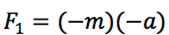is positive, and a force with an opposite acceleration and positive mass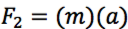is also positive, the second force adds to the acceleration of the first, even though the objects are accelerating in opposite directions.

The only way in which the particle with negative mass can be slowed down is if a force is applied from another object with negative mass (similar to how two objects with positive mass interact), or experiences a force from a positive mass that is accelerating the same direction as it is. Given a negative mass for Newton’s second law, no contradiction seems to arise, nor is there a need to create a new equation to describe the motion of objects with negative mass that travel beyond light speed.

Momentum:

The equation for force can be integrated to find the equation of momentum and a similar definition for objects with positive and negative mass arises. However, the momentum involved below will be for objects with both positive and negative mass which are not near the speed of light. The relativistic equations for momentum are found in Parts 1 and 2 of this paper. The classic Newtonian equation for momentum is:

p = mv (Proposed addition: m can either be negative or positive)

While the relativistic definition is:

In both cases, having a negative mass will result in an increase in velocity when impulse is applied in a direction opposite to its movement. The relative definition of momentum also works for objects with negative mass, with the exception of when the velocity of the object is the speed of light (in which case the mass is zero).

Relativistic Energy in Terms of Momentum:

Given: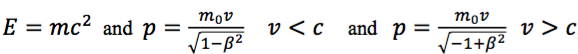, two definitions of energy can be derived, one for positive mass (and velocities less than that of light), and one for negative mass (and velocities greater than that of light)

The derivation starts with the pc combination used to describe a particles momentum, and relate it to the energy of the particle.

Above is the relativistic definition of energy in terms of momentum, in the current model with the current Lorentz factor used during derivation. Now the same derivation will be done, but with the new Lorentz transformation, which should work for objects with negative mass.

As seen in this derivation, this new description of energy will work for objects exceeding the speed of light, and for objects with negative mass, whereas the previous model only worked for objects traveling at speeds less than that of light. However, the two equations are not interchangeable, as one will not work in the constraints of the other. The two are related by the same factor (i) discussed when deriving a new gamma value. In all cases, energy remains positive, hence, the addition of negative mas and faster than light particles does not contradict this aspect of the current model. The new definitions of momentum, force, and energy are to be additions to the current model which work in the cases where an object with negative mass is traveling beyond the speed of light

Relationship between the two Energy Equations:

As seen before with the relationship between the two gammas, the energy equations also demonstrate this parallel structure through the multiplication of the current equation with (i) to get the new equation for objects with negative mass and velocity greater than light.

Gravity:

Gravity will not be discussed in this paper, as it deals with non-Newtonian physics, and requires a discussion of general relativity. The main focus of this paper is on special relativity, and possible ways in which the speed of light is exceeded. The hypothesis proposed is that an object with negative mass will repel something with normal mass, this will be discussed in a later paper on expanding general relativity.

Conclusion:

The overall premise of this paper pivots on the assumption that there are particles with negative mass which travel beyond the speed of light, which are yet to be detected. With this assumption, it is seen that a new gamma value can be derived, and this value is an exact parallel to the current model of special relativity. The fact that it mirrors the current model, and does not contradict its assumptions, shows that it might have some merit. Moreover, the following conclusions about the behavior of all particles can be described as: no particle with positive mass can reach the speed of light, a particle with no mass can travel at the speed of light, and a particle with negative mass always travels faster than the speed of light. This not only complies to the law that the speed of light cannot be attained, but also encompasses all possible combinations of mass and velocity (both real and theoretical). Also, the possibilities of negative mass, and the theoretical behavior of such matter was discussed, although gravity was not addressed (this will be done in a later paper). The addition of negative mass to the current models of special relativity and Newtonian mechanics does not seem to contradict the models, but provides an addition to them for special cases (in this case negative mass and speeds beyond that of light). With the new Newtonian equations, it is predicted that a particle with negative mass will accelerate to a greater speed when a force is applied to slow it down. Therefore, it becomes impossible to slow it down to the speed of light, as it would require an infinite amount of energy. This is a direct parallel to the way in which a particle with mass behaves when trying to reach the speed of light. Lastly, the additions to two of the most prominent models in physics proposed in this paper are essential in understanding the nature of dark matter and energy. With these additions, it becomes easier to understand what dark matter might be, as it exhibits negative mass like properties. Whether or not this dark matter travels faster than light is still a mystery, but the expanded models of SR and Newtonian mechanics might be useful in understanding the physics of this strange matter.

Acknowledgments:

My physics teacher Mrs. Anton, who has reviewed and critiqued my paper, and suggested I explore relativistic energy. Physics teacher Mr. Dobson, who has helped me look into possible contradictions in my paper. Physics and astronomy teacher Mr. Brocketti, who was the first to listen to my proposal for time dilation beyond light speed. My math teacher Dr. Ricketts. (All of them teach at McLean High school)

Sources

• Derivation of Time Dilation. (n.d.). Retrieved November 22, 2018, from https://users.sussex.ac.uk/~waa22/relativity/Complete_Derivation_files/derivation.pdf
• Desmos graph. (n.d.). Retrieved November 25, 2018, from https://www.desmos.com/calculator
• Etkina, E., Gentile, M., & Heuvelen, A. V. (2014). College physics. Boston: Pearson.